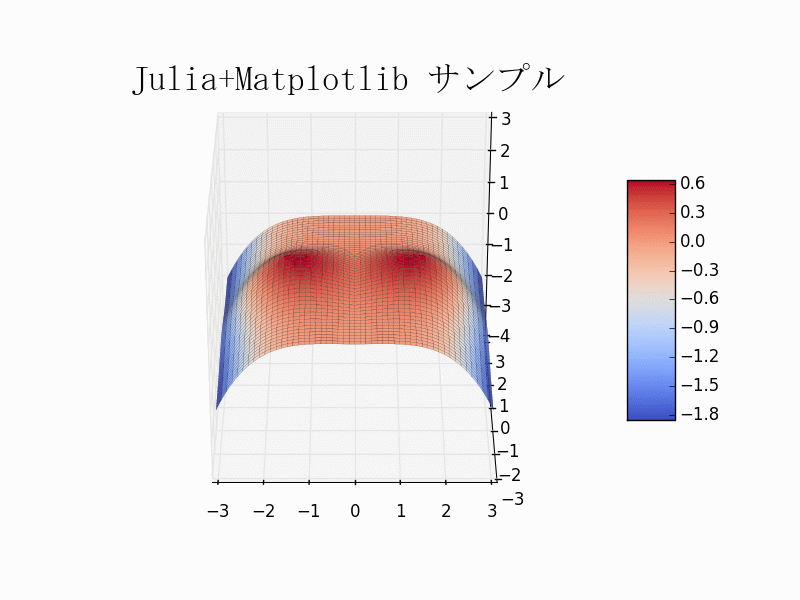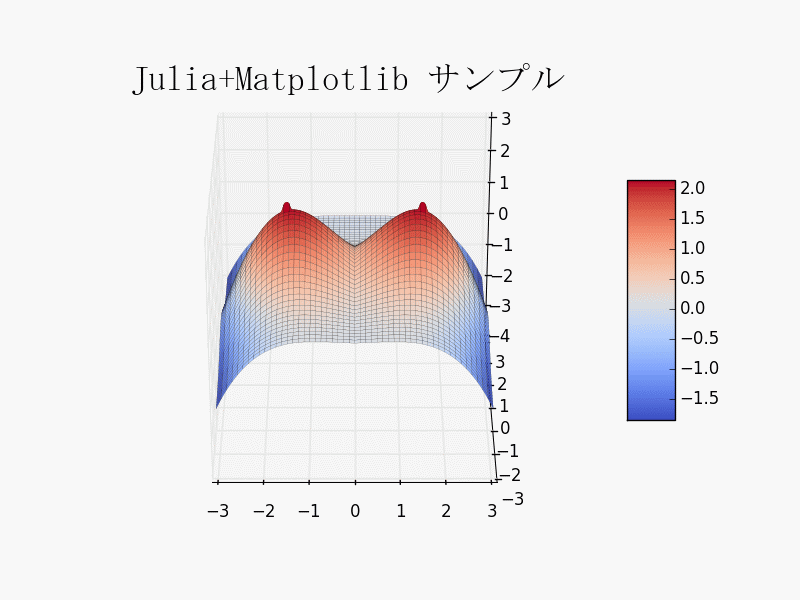# [PYTHON] Draw a "breast curved surface" in a 3D graph (2)

"Draw a graph in the programming language Julia" I succeeded in moving the sample for the time being, but the data created by my own program (in Julia language) I decided to find out how to draw a graph using.

This time, we will conduct an experiment to enlarge "boobs" by referring to "Length calculation by boobs curved surface equation". I tried to. The calculation result obtained by Julia is displayed as a graph by Matplotlib. (Please create an environment and move it)

• When saving the source code, the character encoding should be utf-8.
• To launch the sample, double-click Oppai2.jl (Julia source code) from Explorer.
• Increasing ALPHA in the source code will increase the "boobs". Increasing BETA will extend the "nipple".
• A file called "Oppai2.png " will be created in the current directory.

Note: For the environment construction, refer to "Drawing a graph in the programming language Julia". Added steps 4, 6 and 11 on 2014/07/04. ** If you have built the environment by looking at the above article before 2014/07/04, please perform additional steps 4, 6 and 11. **Thank you.

↓ Example of \$ ALPHA = 1 \$. "Breasts" are small. Drag the left button to rotate the 3D graph. You can zoom in / out by dragging the right button.↓ Example of \$ ALPHA = 3 \$. "Breasts" are big. Drag the left button to rotate the 3D graph. You can zoom in / out by dragging the right button.#### `Oppai2.jl`

``````
# -*- coding: utf-8 -*-

using PyPlot

using PyCall
@pyimport matplotlib.cm as cm
@pyimport matplotlib.font_manager as fm
@pyimport matplotlib.pyplot as plt
@pyimport numpy as np

ALPHA = 1.0
BETA  = 1.0
GAMMA = 1.0 / ALPHA

function bust(x, y)
ALPHA * (6 * exp(-((2 / 3 * abs(x) - 1) ^ 2 + (2 / 3 * y) ^ 2) - 1 / 3 * (2 / 3 * y + 0.5) ^ 3)
+ BETA * 2 / 3 * exp(-2.818 ^ 11 * ((abs(2 / 3 * x) - 1) ^ 2 + (2 / 3 * y) ^ 2) ^ 2)
- GAMMA * (2 / 3 * x) ^ 4) / 8
end

fig = plt.figure()
ax = fig[:gca](projection="3d")

nx = 201
ny = 201
x = linspace(-3, 3, nx)
y = linspace(-3, 3, ny)
z = zeros(Float64, length(y), length(x))

for iy = 1:length(y)
for ix = 1:length(x)
z[iy, ix] = bust(x[ix], y[iy])
end
end

x, y = np.meshgrid(x, y)
sf = ax[:plot_surface](x,y,z,rstride=4,cstride=4,cmap=cm.coolwarm,linewidth=0.1,antialiased=true)
fig[:colorbar](sf,shrink=0.5,aspect=5)

ax[:set_zlim](-4,3)
ax[:view_init](0,-90)

@windows? (
begin
#fp = fm.FontProperties(fname="C:\\WINDOWS\\Fonts\\msgothic.ttc")
fp = fm.FontProperties(fname="C:\\WINDOWS\\Fonts\\msmincho.ttc")
plt.title("Julia+Matplotlib sample", fontproperties=fp, fontsize=25)
end
: begin
plt.title("Julia+Matplotlib sample", fontsize=25)
end
)

plt.savefig("Oppai2")
plt.show(block=true)
``````